# Optimization

## Notation

•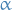- learning rate
•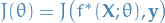- cost of using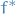to approximate the real function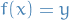for observed input-output pairs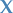and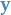•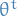- approx. value for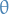after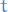iterations

## First-order

### Overview

First-order optimization techniques only take into account the the first-order derivative (the Jacobian).

These kind of methods are of course usually slower than the second-order techniques, but posess the advantage of being much more feasible for high-dimensional data.

Consider some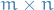weight matrix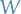. Taking the derivative of some function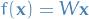gives us a Jacobian matrix ofdimensions, i.e.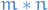derivatives. If we want the Hessian, we then have to take the partial derivatives of all the combinations of the Jacobian, givig us a total of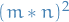partial derivatives to compute. See the issue?

#### Motivation

• Finding minimum of some arbitrary function without having to compute the second-order derivative
• Preferable iterative method, allowing incremental improvements

#### Definition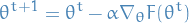1

where

•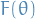is some arbitrary function
•is the learning-rate

Typically in machine learning we want to minimize the cost of some function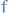over some parameters, which we might denote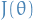(in my opinion, more precisely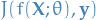). Usually we don't have access to the entire functionbut samples of input-output pairs. Therefore we cannot compute the full gradient ofand need to somehow approximate the gradient. And so Batch Gradient Descent arises!

#### Motivation

• Perform gradient descent without having to know the entire function we're approximating

#### Definition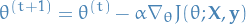2

where the gradient now is the average over all observations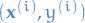#### Issues

• Expensive to compute average gradient across entire set of observations
• Convergence tends to be slow as some data where our prediction is way off might be "hidden" away in the average

#### Motivation

• Computing the full approximation to the gradient in Batch Gradient Descent is expensive
• Also want to address the issue of potentially "hidden" away mistakes we're making

#### Definition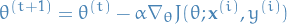3

where now the gradient is compute from a single observation.

#### Issues

• Usually quite unstable due to high variance
• Can be mitigated by decreasing the learning rate as we go, but this has the disadvantage of vanishing gradient

These days, when people refer to Stochastic Gradient Descent (SGD) they most of the time mean Mini-batch Gradient Descent. It's just generally much better.

#### Motivation

• Reduce instability (variance) of Stochastic Gradient Descent
• Improve the slow & expensive Batch Gradient Descent
• Make use of highly optimized matrix-operations

#### Definition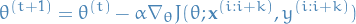4

where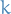is the size of the mini-batch.

#### Issues

• Choosing proper learning-rateis hard
• SGD only guarantees convergence local minimas for non-convex functions, and can easily be "trapped" in these local minimas
• In fact, saddle-points are argued to be a larger problem, as the gradient is zero in all dimensions, making it hard for SGD to "escape" / "get over the edge and keep rolling"

### Momentum SGD

#### Motivation

• If we keep seeing that is a "good thing" to update in some direction, then it would make sense to perform larger updates in this direction

#### Definition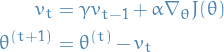5

I really suggest having a look at Why Momentum Really Works by Gabriel Goh. It provides some theoretical background to why this simple addition works so well, in combinations with a bunch of visualizations to play with.

#### Issues

• We have tendency to "overshoot" since we now have this "velocity" component, leading to oscillations around the point of convergence instead of reaching it and staying there. This is especially an issue for non-convex functions as we might actually overshoot the global minima.

### Nestorov Momentum SGD

#### Motivation

• Better control the overshooting of Momentum SGD

#### Definition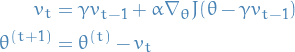6

Here we approximate the next value of(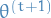) by evaluating the gradient at the current point,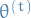, minus the momentum-term we'll be adding,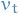, to obtain. In this way we get a certain control of the step we're taking by "looking ahead" and re-adjusting our update.

If the update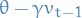is indeed a "bad" update, then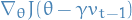will point towardsagain, correcting some of the "bad" update.

Sometimes people swaps the + sign in the update, like in the paper I'm about to suggest that you have a look at:

Have a look at Ilya Sutskever's thesis in section 7.2 where you get a more mathematical description of why this is a good idea.

#### Motivation

• We would like to adapt our updates to each individual parameter to perform larger or smaller updates depending on the importance of the parameter
• Say you barely see this one parameter in your updates, but then suddenly you look at an observation where this parameter has a significant impact. In this case it would make sense to perform a greater-than-normal update for this parameter.
• We still don't like the fact that all previous methods makes us have to manually tune the learning rate#### Definition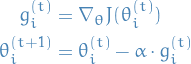7

where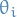denotes the ith component of.

Or better: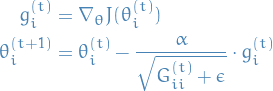8

where

•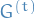is a diagonal matrix where each diagonal element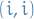is the sum of the squares of the gradients w.r.t.up to•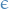is a smoothing term that avoids zero-division (usually on the order of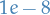)

Finally to make use of efficient matrix-operations: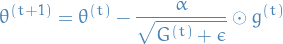9

#### Issues

• Accumulation of the squared gradient in the denominator means that eventually the denominator will so large that the learning-rate goes to zero

### RMSProp

#### Motivation

• Fix the issue of the too aggressive and monotonically decreasing learning rateof Adagrad